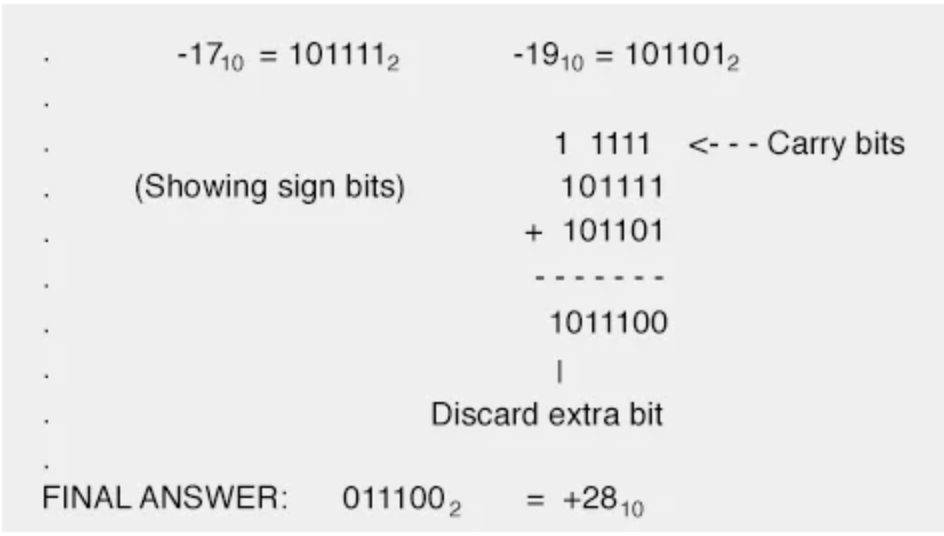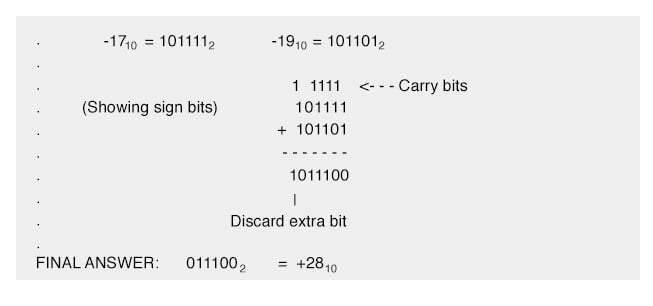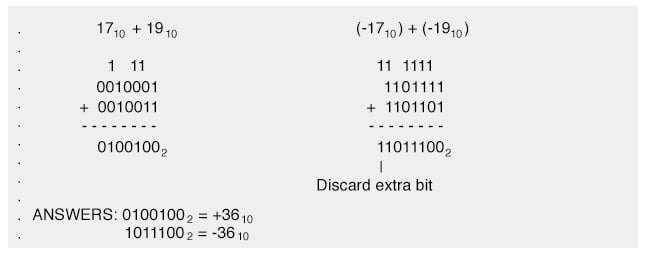## When does Binary Overflow occur?

Overflow occurs when the magnitude of a number exceeds the range allowed by the size of the bit field.

# Binary Overflow

## Chapter 2 - Binary Arithmetic

One caveat with signed binary numbers is that of overflow, where the answer to an addition or subtraction problem exceeds the magnitude which can be represented with the allotted number of bits. Remember that the place of the sign bit is fixed from the beginning of the problem.

With the last example problem, we used five binary bits to represent the magnitude of the number, and the left-most (sixth) bit as the negative-weight, or sign, bit. With five bits to represent magnitude, we have a representation range of 25, or thirty-two integer steps from 0 to maximum.

This means that we can represent a number as high as +3110 (0111112), or as low as -3210 (1000002).

### Restrictions of the Six-bit Number Field

If we set up an addition problem with two binary numbers, the sixth bit used for sign, and the result either exceeds +3110 or is less than -3210, our answer will be incorrect. Let’s try adding 1710 and 1910 to see how this overflow condition works for excessive positive numbers:The answer (1001002), interpreted with the sixth bit as the -3210 place, is actually equal to -2810, not +3610 as we should get with +1710 and +1910 added together! Obviously, this is not correct. What went wrong?

The answer lies in the restrictions of the six-bit number field within which we’re working Since the magnitude of the true and proper sum (3610) exceeds the allowable limit for our designated bit field, we have an overflow error.

Simply put, six places doesn’t give enough bits to represent the correct sum, so whatever figure we obtain using the strategy of discarding the left-most “carry” bit will be incorrect.

A similar error will occur if we add two negative numbers together to produce a sum that is too low for our six-bit binary field. Let’s try adding -1710 and -1910 together to see how this works (or doesn’t work, as the case may be!):The (incorrect) answer is a positive twenty-eight. The fact that the real sum of negative seventeen and negative nineteen was too low to be properly represented with a five bit magnitude field and a sixth sign bit is the root cause of this difficulty.

### Using the Seventh Bit for a Sign Bit

Let’s try these two problems again, except this time using the seventh bit for a sign bit, and allowing the use of 6 bits for representing the magnitude:By using bit fields sufficiently large to handle the magnitude of the sums, we arrive at the correct answers. In these sample problems we’ve been able to detect overflow errors by performing the addition problems in decimal form and comparing the results with the binary answers.

For example, when adding +1710 and +1910 together, we knew that the answer was supposed to be +3610, so when the binary sum checked out to be -2810, we knew that something had to be wrong. Although this is a valid way of detecting overflow, it is not very efficient.

After all, the whole idea of complementation is to be able to reliably add binary numbers together and not have to double-check the result by adding the same numbers together in decimal form! This is especially true for the purpose of building electronic circuits to add binary quantities together: the circuit has to be able to check itself for overflow without the supervision of a human being who already knows what the correct answer is.

What we need is a simple error-detection method that doesn’t require any additional arithmetic. Perhaps the most elegant solution is to check for the sign of the sum and compare it against the signs of the numbers added.

Obviously, two positive numbers added together should give a positive result, and two negative numbers added together should give a negative result. Notice that whenever we had a condition of overflow in the example problems, the sign of the sum was always opposite of the two added numbers: +1710 plus +1910 giving -2810, or -1710 plus -1910 giving +2810.

By checking the signs alone we are able to tell that something is wrong. But what about cases where a positive number is added to a negative number? What sign should the sum be in order to be correct. Or, more precisely, what sign of sum would necessarily indicate an overflow error?

The answer to this is equally elegant: there will never be an overflow error when two numbers of opposite signs are added together! The reason for this is apparent when the nature of overflow is considered. Overflow occurs when the magnitude of a number exceeds the range allowed by the size of the bit field.

The sum of two identically-signed numbers may very well exceed the range of the bit field of those two numbers, and so in this case overflow is a possibility. However, if a positive number is added to a negative number, the sum will always be closer to zero than either of the two added numbers: its magnitude must be less than the magnitude of either original number, and so overflow is impossible.

Fortunately, this technique of overflow detection is easily implemented in electronic circuitry, and it is a standard feature in digital adder circuits: a subject for a later chapter.

RELATED WORKSHEETS:

• Share
Published under the terms and conditions of the Design Science License